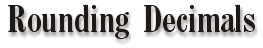Find the decimal place you are asked to round to: -If the number is greater than or equal to a five, round up. -If the number is less than five, leave it alone. -Cut off any remaining decimal places further to the right.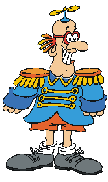Example: Round .123456 to the nearest thousandth. The 3 is in the thousandths place. Look to the right of the 3 and find a 4. Our result is .123. Example: Round .599 to the nearest hundredth. The 9 is in the hundredths place. Look to the right and find a 9. Our result is .60.

 Note: When dealing with "real life" problems, you must realize how many decimal places you need to use so that the problem makes sense. For example, dollars and cents will only be rounded to 2 places, because there are 99 cents in a dollar.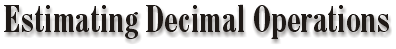Examples:

Batting average is rounded to the thousandth place.
Earned run average is rounded to the nearest hundredth.
Dollar amounts are rounded to the nearest one or tenth.

 To help Babe figure out this problem, you will have to use estimation.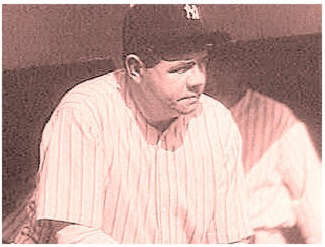Hmm... My batting average is .313. That pitcher's E.R.A. is 6.87. And the 5 hot dogs I just ordered cost a buck thirty-five each. If I want to tip the vendor 2 dollars, how much should I give her?

 Addition & Subtraction: \$4.37 + \$0.99 + \$1.75 + \$2.53 -\$1.90 = \$4.50 + \$1.00 + \$2.00 + \$2.50 - \$2.00 = \$8.00 Note: When estimating prices, especially at the grocery store, it is better to over estimate rather than underestimate. Example: \$2.65 should be estimated to \$3.00 rather than \$2.50 Multiplication: Giant Oranges are \$0.85, each so you buy 5. Your bill will be less than \$5.00 since you estimate each orange as costing a \$1.00 and 5 x \$1.00= \$5.00. Division: You buy 6 drinks for you and your friends, and the bill is \$5.80. How much per drink? About \$1.00 because \$5.80 is about \$6.00 and \$6.00 divided by 6 is \$1.00.

 How much should Babe give the vendor? 5 at \$1.35 and a \$2.00 tip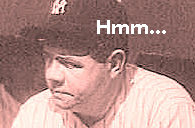Decimals Main